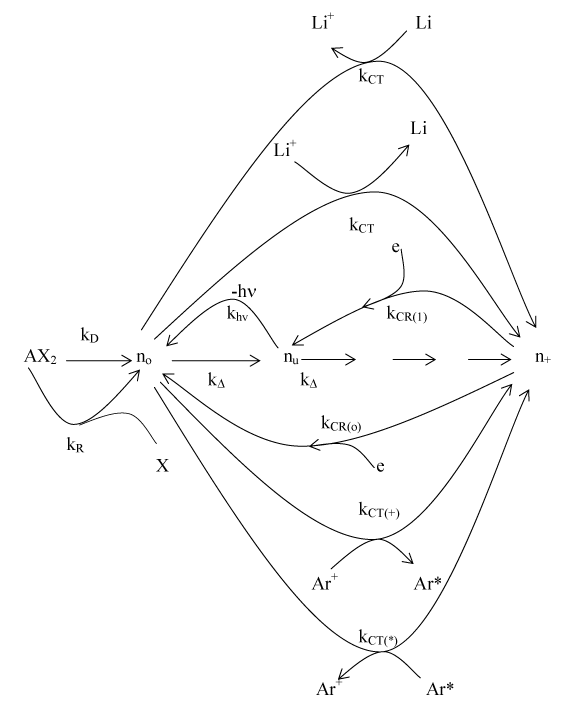Figure 2: Schematic representation of the proposed model  n denotes number density, the subscripts o, u, +, AX2, X, mo, and m+ denote analyte ground state, analyte excited state, analyte ion, analyte salt, counter atom, ground state interferent atom and interferent ion, respectively; Ar(*) and Ar(+) denote argon excited state and ions, respectively; the prime in nu’, ne’ and nx’ denotes quantities measured in the presence of the interferent; kDM is the rate constant for thermal dissociation, kΔ is the rate constant for thermal excitation from ground state; kCR(0) is the rate constant for ion-electron collisional recombination to the ground state; kCR(1) is the rate constant for ionelectron collisional recombination to the first excited state; kCT and kCT’ are the rate constants for collisional charge transfer involving Li species; kCT(*) and kCT(+) are the rate constants for collisional charge transfer involving argon species; kR is the rate constant for collisional recombination of analyte and counter atoms.
Goto home»